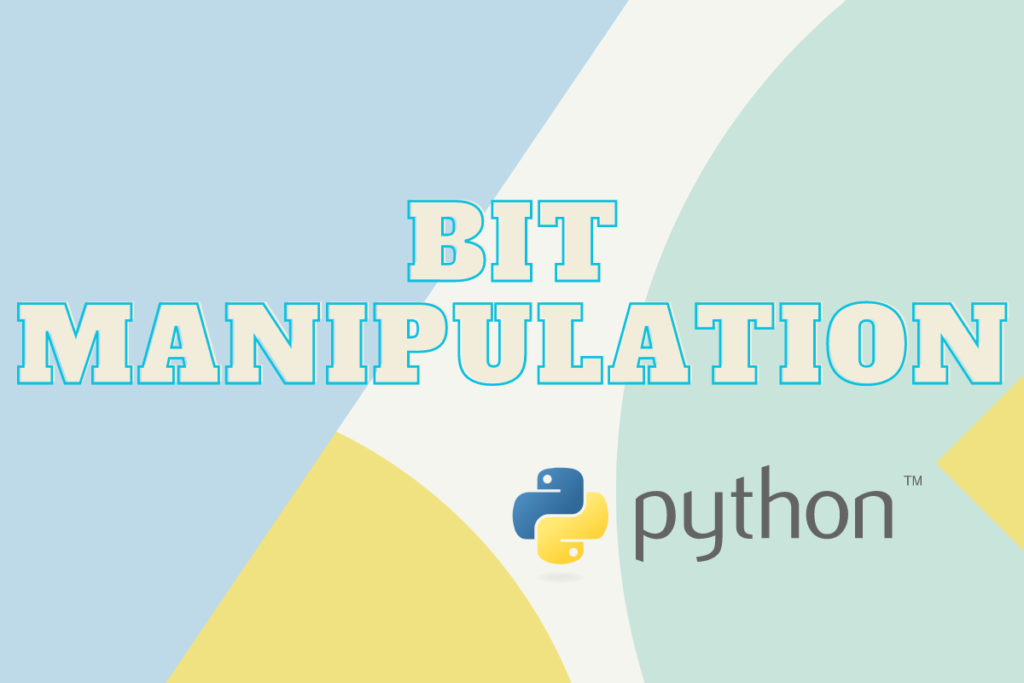# Python Bit Manipulation and Masking TechniquesA bit is the fundamental block for building any programming language. In this article we shall explore the ways that Python offers to manipulate and put some fancy masks on those bits.

## Understanding Bit Manipulation in Python

Bit manipulation is a method to catalyse the speed at which the arithmetic operations are carried out by considering a number to be a string of bits. These bits are then shifted hither & thither to manipulate their sequence and provide the desired result. This technique is used in a variety of problems to get the solution in the easiest way.

Also check: 5 NumPy Bitwise Operations to know

## Introducing Bit Masking in Python

Similar to our face masks, bit masks are used to cover a value. One can then choose to keep that value for secretive purpose or modify it as well. This masked value gains some importance to serve as a representative for the subsets whilst carrying out several bitwise operations. Now that we know what manipulating and masking a bit is, let us now understand how it can be carried out & the resources needed for that.

## Python Bitwise Operators

Listed below are some of the operators in Python that aids in juggling around the bits. Let us have a look at how the 0’s and 1’s are wiggled around using these operators.

• AND  &
• OR  |
• NOT  ~
• XOR  ^
• LEFT-SHIFT  <<
• RIGHT-SHIFT  >>

### Python AND operator (&)

Only when both bits are ‘1’, then the output shall considered to be true by the AND operator and ‘1’ is returned, else 0 would be returned instead. Given below are the binary equivalents of 6 and 2 alongside the corresponding results when AND operator is applied.

```6 = 110
2 = 010
--------------
2 = 010
```
```print(6 & 2)
```

When running the above code, the result shall be ‘2’.

### Python OR operator (|)

This operator is a bit relaxed when compared to AND and shall return ‘1’ even if any one of the bits in the given inputs is ‘1’. But in cases where both are 0’s, then it is considered as false and ‘0’ is returned as the output.

```6 = 110
2 = 010
--------------
6 = 110
```
```print(6 | 2)
```

When the above code is run, it returns ‘6’ as the result since the binary equivalent after running through the OR operator is same as ‘6’.

Also read: The += Operator In Python – A Complete Guide

### Python NOT operator (~)

Imagine someone who always insists on the opposite of what you say. The same goes for the NOT operator as well. It shall invert the value (i.e) negate the given input which in this case would be to turn 0’s to 1’s and vice versa.

While numbers can be both positive and negative, the conversion of the binary equivalents into their decimal counterparts is carried by a technique known as the complement of 2’s.

To put in simple terms for all those binary representations where the leading bits are formed by 1’s, their decimal equivalents are considered to be negative.

### Python XOR operator (^)

This operator returns 1 when one of the input bits is 1, while if both the inputs are identical (i.e) both are 0’s or both are 1’s, then it shall return ‘0’.

```13 = 1101
5   = 0101
--------------
8  = 1000
```
```print(13 ^ 5)
```

Running the above code shall return ‘8’ as the result.

### Python LEFT-SHIFT Operator(<<)

The LEFT-SHIFT Operator can be used to shift the bits towards the left for the specified number of places thereby converting it into the binary equivalent of the desired decimal. Let us have a look at the below examples in which we have going to shift the bits of binary 5 to two places.

```print(5 << 2)
```

Running the above code shall return ‘20’ as the result. Wanna know why?

Binary equivalent of 5 is 0000 0101, which after shifting 2 places to the left becomes 0001 0100 which represents the decimal 20.

Another interesting feature about the LEFT-SHIFT operator is to deduce the powers of 2. For instance, if one ought to find the nth power of 2, all that needs to be done is to shift 1 to the number of times the power as shown below.

### Python RIGHT-SHIFT Operator(>>)

This is the converse of the LEFT-SHIFT operator where the bits are moved towards the right to the specified number of places from their existing position. In the below example, let us carry out right shifting for 5 by moving it to 2 places.

```print(5 >> 2)
```

Running the above code shall return ‘1’ as the result. Wanna know why? Binary equivalent of 5 is 000 0101, which after shifting 2 places to the right becomes 0000 0001 which represents the decimal 1.

## Conclusion

We’ve now explored various Python techniques for bit manipulation and masking. Python offers a range of bitwise operators that enable you to control and manipulate bits in numbers easily.

As you continue your Python journey, consider how these techniques can improve the efficiency of your code. What new applications can you envision using these bit manipulation techniques in Python?